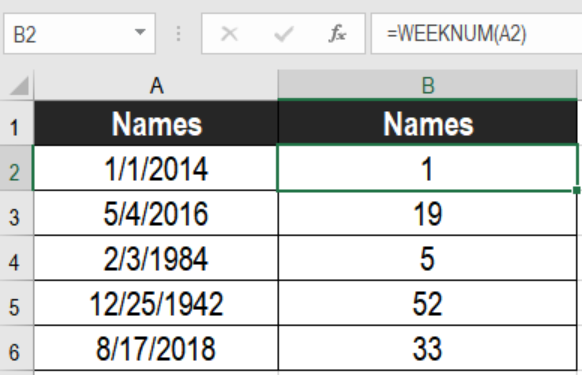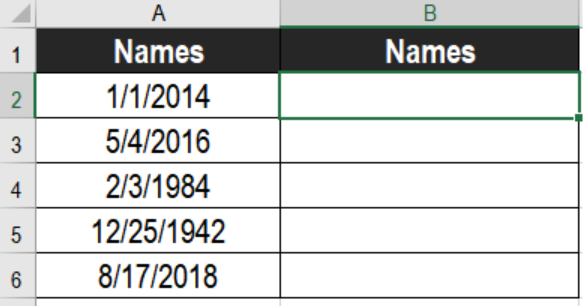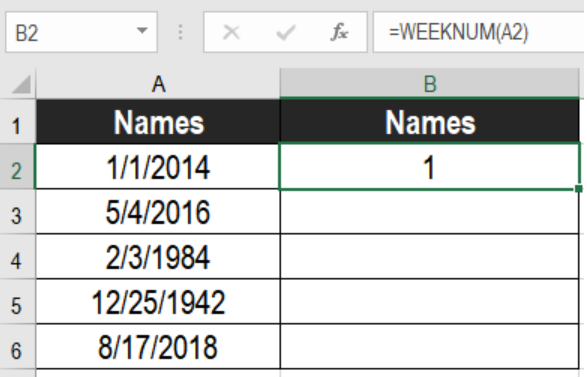Get instant live expert help with Excel or Google Sheets“My Excelchat expert helped me in less than 20 minutes, saving me what would have been 5 hours of work!”

#### Post your problem and you’ll get Expert help in seconds.

Your message must be at least 40 characters
Our professional Expert are available now. Your privacy is guaranteed.

# Excel WEEKNUM Function

The WEEKNUM function in Excel is one of the major functions used by financial analysts. It helps us to find out the week number from a given date. This function tells us which week number (1-54) in the year a date belongs to. While working on financial statements, it is important to count the days and weeks. That’s when the WEEKNUM function shines. In this tutoria, we will learn how to use the WEEKNUM function in Excel.Figure 1. Example of how to use the Excel WEEKNUM Function

## Syntax

`=WEEKNUM (serial_num, [return_type])`

## Arguments

• serial_num
This argument is required. It is the date which you want to find the number of week.
• return_type
This is an optional argument. It specifies the system of weekday numbering. It helps to select the starting weekday and ending weekday. The default value is 1 which represents Sunday. You can change this value to start the week count from another day –

2 – week starts with Monday.

12– week starts with Tuesday.

## Process

According to the WEEKNUM function, Weeks start on Sunday. It starts counting with the week containing January 1st. Next, it counts the week number till the desired date is reached.

Weeknum has another way of calculating the number of week. The week having the first Thursday of the year is considered the first week of the year. WEEKNUM assigns it as week 1. The roots of this system matches with ISO 8601. It is also known as the European week numbering system.

## Setting Up Data

The following example contains some sample dates. Column A has these dates.Figure 2. The Data Set

To find out the week number from these dates using the Excel WEEKNUM function

• We need to left click on cell B2 with our mouse.
• Then, we have to assign the formula `=WEEKNUM(A2)` to B2.
• Press Enter.Figure 3. Applying the Formula

• Drag the formula from cells B2 to B6. We can use the fill handle on the bottom right to apply the formula to the entire column.

This will make column B show the week numbers of the dates.

## Notes

1. Excel returns a #VALUE! error if serial_num is a numeric value. It returns a #NUM! error if the current date-base value makes serial_num out of range.
2. Excel returns a #NUM! error if return_type is out of range.

Most of the time, the problem you will need to solve will be more complex than a simple application of a formula or function. If you want to save hours of research and frustration, try our live Excelchat service! Our Excel Experts are available 24/7 to answer any Excel question you may have. We guarantee a connection within 30 seconds and a customized solution within 20 minutes.

Solution examplesI need a formula that has these 2 rules. If letter was rec'd between 1st and 15th = 1st of the month following letter rec'd date. If letter was rec'd between 16thst and end of the month= 1st of the 2nd month following letter rec'd date.
Solved by V. F. in 20 minsI need a column to total (Hours)(85) + (Travel)(.55) per line
Solved by F. Q. in 20 minsTrying to figure out what is wrong with my formula in Oct-ZD tab in column AY and BG.
Solved by O. Q. in 8 minsSo I have used vlookup to find the price of a product on a different sheet and then I have tried to times that by a quantity. e.g. =vlookup(d4,table35,2,false)*e4 This is the formula I typed in which worked for another list I did but for some reason it's not working now even though everything is practically the same, apart from the contents. Could you help?
Solved by B. U. in 60 minsI have a column containing date and time data together and i need to isolate the time data. When I tried to use text to column, it changed all the times to AM. There is an extremely large amount of data - over 550,000 rows so manually changing it won't work. The file is too large to attach.
Solved by S. E. in 40 mins